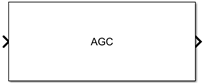AGC

Library

RF Impairments Correction

•Description

The automatic gain controller (AGC) block adaptively adjusts its gain to achieve a constant signal level at the output.

Parameters

Step size

Specify the step size for gain updates as a double-precision or single-precision real positive scalar. The default is 0.01.

If you increase Step size, the AGC responds faster to changes in the input signal level. However, gain pumping also increases.

Desired output power (W)

Specify the desired output power level as a real positive scalar. The power level is specified in Watts referenced to 1 ohm. The default is 1.

Averaging length

Specify the length of the averaging window in samples as a positive integer scalar. The default is 100.

Note

If you use the AGC with higher order QAM signals, you might need to reduce the variation in the gain during steady-state operation. Inspect the constellation diagram at the output of the AGC and increase the averaging length as needed. An increase in Averaging length reduces execution speed.

Maximum power gain (dB)

Specify the maximum gain of the AGC in decibels as a positive scalar. The default is 60.

If the AGC input signal power is very small, the AGC gain will be very large. This can cause problems when the input signal power suddenly increases. Use Maximum power gain (dB) to avoid this by limiting the gain that the AGC applies to the input signal.

Enable output of estimated input power

Select this check box to provide an input signal power estimate to an output port. By default, the check box is not selected.

Simulate using

Select the simulation mode.

Code generation

On the first model run, simulate and generate code. If the structure of the block does not change, subsequent model runs do not regenerate the code.

If the simulation mode is Code generation, System objects corresponding to the blocks accept a maximum of nine inputs.

Interpreted execution

Simulate model without generating code. This option results in faster start times but can slow subsequent simulation performance.

Algorithms

This block implements the algorithm, inputs, and outputs described on the comm.AGC reference page. The object properties correspond to the block parameters.

Examples

To open these examples, enter the example names at the MATLAB command prompt:

• doc_agc_plot_step_size — Plots the effect of step size on AGC performance.

• doc_agc_plot_max_gain — Shows how the maximum gain affects the ability of the AGC to reach its target output power.